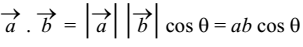# Scalar or Dot Product

The scalar product of two vectors is a scalar quantity. Therefore, the product is called scalar product.### Sign of Dot Product

The dot product may be positive or negative or zero.

1. If the angle between the two vectors is acute (0° < θ < 90°) then cos θ is positive. In this case, dot product is positive.

2. If the angle between the two vectors is obtuse (90° < θ < 180°) then cos θ is negative. In this case, dot product is negative.

3. If the angle between the two vectors is 90° (θ = 90°) then cos θ = 0. In this case, dot product is zero.